makewavelets
Save wavelet decomposition results to the workfile.
Syntax
Series View: series_name.makewaveobj(options)
Options
Basic Options

 proc=arg (default=“decomp”) Wavelet analysis type: “decomp” (transform), “anova” (variance decomposition), “outlier” (outlier detection), “threshold” (threshold - denoising).
Wavelet Transform Options

 transform=arg (default=“dwt”) Wavelet transform type: “dwt” (discrete wavelet transform – DWT), “modwt” (maximum overlap DWT – MODWT), “mra” (DWT multiresolution analysis – DWT MRA), or “momra” (MODWT MRA).Note that when performing DWT or MRA, if the series length is not dyadic, a dyadic fix may be set with the “fixlen=” option fixlen=arg (default=“mean”) Fix dyadic lengths in DWT and MRA transforms: “zeros” (pad remainder with zeros), “mean” (pad remainder with mean of series), “median” (pad remainder with median of series), “shorten” (cut series length to dyadic length preceding series length). maxscale=integer (default = max possible) Maximum scale for wavelet transform. The max possible is obtained as follows. Let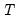denote the series length and decomposeinto its dyadic component and a remainder: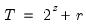,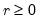. The default maxscale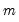is then set with the following rules:DWT: (1) if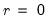then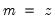, otherwise (2) if expanding the series,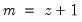and (3) if contracting the series.MODWT:. filter=arg (default=“h”) Wavelet filter class: “h” (Haar), “d” (Daubechies), “la” (least asymmetric).If “filter=h” or “filter=la”, the filter length may be specified using “flen=”.Wavelet filter boundary conditions are specified using the “bound=” option flen=integer Wavelet filter excess length as an even number between 2 and 20.For use when “filter=d” (default= 4) or “filter=la” (default=8). bound=arg (default = “p”) Filter boundary handling: “p” (periodic), “r” (reflective). basename=arg (default is series name) Basename for output:1. When “transform=dwt” or “transform=modwt”:Wavelet coefficient output will be saved in vectors with names given by basename followed by “_w#” where “#” is the scale level.Scaling coefficient output will be saved in vectors with names given by basename followed by “_v#” where “#” is the scale level.2. When “transform=mra” or “transform=momra”:Detail output will be saved in vectors with names given by basename followed by “_d#” where “#” is the scale level.Smooth output will be saved in vectors with names given by basename followed by “_s#” where “#” is the scale level.
Wavelet Variance Decomposition Options

 variance=arg (default = “nobias”) Wavelet variance type: “nobias” (unbiased variance), “bias” (biased variance). ci=arg (default = “none”) Confidence interval type: “none” (no CIs computed), “gauss” (asymptotic normal), “chisq” (asymptotic chi-square), “blimit” (band-limited). cilevel=arg (default = 0.95) Confidence interval coverage as a number between 0 and 1. transform=arg (default=“dwt”) Wavelet transform type: “dwt” (discrete wavelet transform – DWT), “modwt” (maximum overlap DWT – MODWT). Note that when performing DWT, if the series length is not dyadic, a dyadic fix may be set with the “fixlen=” option fixlen=arg (default=“mean”) Fix dyadic lengths in DWT and MRA transforms: “zeros” (pad remainder with zeros), “mean” (pad remainder with mean of series), “median” (pad remainder with median of series), “shorten” (cut series length to dyadic length preceding series length). maxscale=integer (default = max possible) Maximum scale for wavelet transform. The max possible is obtained as follows. Letdenote the series length and decomposeinto its dyadic component and a remainder:,. The default maxscaleis then set with the following rules:DWT: (1) ifthen, otherwise (2) if expanding the series,and (3) if contracting the series.MODWT:. filter=arg (default=“h”) Wavelet filter class: “h” (Haar), “d” (Daubechies), “la” (least asymmetric).If “filter=h” or “filter=la”, the filter length may be specified using “flen=”.Wavelet filter boundary conditions are specified using the “bound=” option flen=integer Wavelet filter excess length as an even number between 2 and 20.For use when “filter=d” (default= 4) or “filter=la” (default=8). bound=arg (default = “p”) Filter boundary handling: “p” (periodic), “r” (reflective). basename=arg (default is series name) Basename for variance output.Variance output will be saved in vector with name given by basename followed by “_var”.Confidence interval output will be saved in matrix with name given by basename followed by “_varci”.
Wavelet Threshold and Outlier Options
 threshtype=arg (default = “soft”) Wavelet threshold type: “hard” (hard thresholding), “soft” (soft thresholding). threshlim=arg (default = “universal”) Wavelet threshold limit type: “universal” (universal), “adaptive” (universal adaptive), “minimax” (minimax), “sureshrink” (SureShrink), “fdr” (false discovery rate).If “threshlim=sureshrink”, the grid length may be specified using “ssglen=”.If “threshlim=fdr”, the significance level may be specified using “fdrsig=” wavevar=arg (default = “gauss”) Wavelet coefficient variance method: “mean” (mean absolute deviation), “gauss” (median absolute deviation with Gaussian adjustment), “median” (median absolute deviation), “meanmedian” (mean median absolute deviation). sslen=arg (default = 10) Grid length used in determining the SureShrink limit. fdrsig=arg (default = .05) Significance level as a number between 0 and 1 for false discovery rate limit determination. transform=arg (default=“dwt”) Wavelet transform type: “dwt” (discrete wavelet transform – DWT), “modwt” (maximum overlap DWT – MODWT). Note that when performing DWT, if the series length is not dyadic, a dyadic fix may be set with the “fixlen=” option fixlen=arg (default=“mean”) Fix dyadic lengths in DWT and MRA transforms: “zeros” (pad remainder with zeros), “mean” (pad remainder with mean of series), “median” (pad remainder with median of series), “shorten” (cut series length to dyadic length preceding series length). maxscale=integer (default = max possible) Maximum scale for wavelet transform. The max possible is obtained as follows. Letdenote the series length and decomposeinto its dyadic component and a remainder:,. The default maxscaleis then set with the following rules:DWT: (1) ifthen, otherwise (2) if expanding the series,and (3) if contracting the series.MODWT:. filter=arg (default=“h”) Wavelet filter class: “h” (Haar), “d” (Daubechies), “la” (least asymmetric).If “filter=h” or “filter=la”, the filter length may be specified using “flen=”.Wavelet filter boundary conditions are specified using the “bound=” option flen=integer Wavelet filter excess length as an even number between 2 and 20.For use when “filter=d” (default= 4) or “filter=la” (default=8). bound=arg (default = “p”) Filter boundary handling: “p” (periodic), “r” (reflective).
Outlier Output Options
 basename=arg (default is series name) Basename for outlier output (when “proc=outlier”).Output will be saved in vectors with name given by basename followed by “_olr#” where “#” is the scale level.
Threshold Output Options
 basename=arg (default is series name) Basename for threshold output (when “proc = threshold”):Fit (signal) output will be saved in vector with name given by basename followed by “_sig_th”.Noise output will be saved in vector with name given by basename followed by “_res_th”.Threshold coefficient output will be saved in vectors with names given by basename followed by “_w#_th” (threshold coefficients), and “_v#” (scaling coefficients) where “#” is the scale level.
Examples
srs.makewaveobj(base=out)
creates vectors OUT_W1, OUT_W2, etc., and OUT_V1, OUT_V2, etc., associated with the wavelet coefficients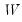and scaling coefficients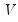from the DWT using a Haar filter.
srs.makewaveobj(transform=modwt, filter=d, flen=6, base=out)
creates vectors OUT_V1, OUT_V2, etc., associated with the scaling coefficientsfrom the MODWT using a Daubechies filter of length 6.
srs.makewaveobj(proc=anova, maxscale=2, fixlen=median, base=out)
creates the vector OUT_VAR with containing variance decomposition per scale using a DWT with maximum scale 2, and a series length adjustment by padding with median values of the series SRS.
srs.makewaveobj(proc=outlier, base=out)
creates vectors OUT_OLR1, OUT_OLR1, etc. with outlier identifiers using a DWT, Haar filter, soft threshold, universal threshold limit, and Gaussian median computation for the wavelet coefficient variance.
srs.makewaveobj(proc=threshold, transform=modwt, threshtype=hard, threshlim=sureshrink, base=OUT)
creates a vector OUT_SIG_TH containing values for the thresholded series, a vector OUT_RES_TH containing noise values from the thresholding procedure, and vectors OUT_W1_TH, OUT_W2_TH, etc. and OUT_W1_TH, CVEC_W2_TH associated with the thresholded wavelet coefficientsand the original scaling coefficientfor the series SRS. The underlying computation uses a MODWT with a Haar filter, a hard threshold with a SureShrink threshold limit.Cross-references
Examples
See “Wavelet Analysis” and “Wavelet Variance Decomposition” for discussion. See also “Wavelet Objects”.